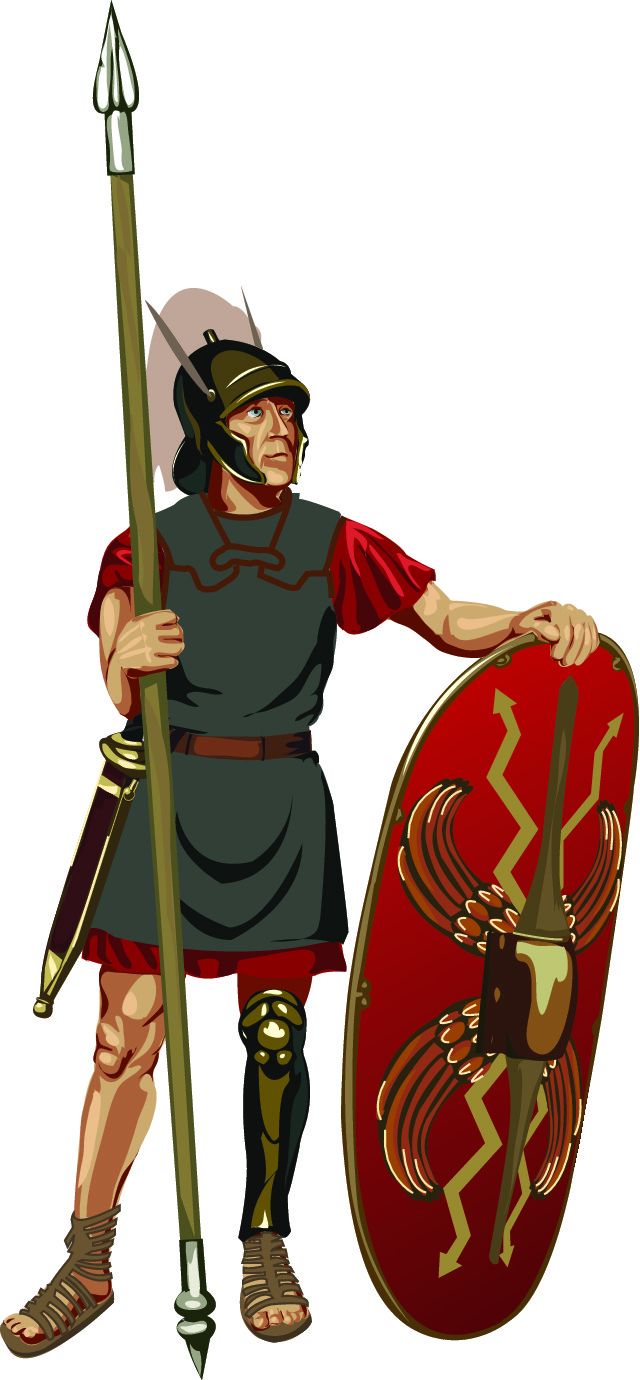# Practise Reading and Writing Roman Numerals up to 100

In this worksheet, students will read and convert numerals to Roman numerals up to 100.Key stage:  KS 2

Curriculum topic:   Number: Number and Place Value

Curriculum subtopic:   Read Roman Numerals (I to C/1 to 100)

Difficulty level:#### Worksheet Overview

Today we use the decimal number system where every number is created using the digits from 0 to 9

However, in Roman times Roman numerals were used.The Romans used these seven different symbols to represent numbers:

I  = 1

V = 5

X = 10

L = 50

C = 100

D = 500

M = 1000

We need to know how to read and write the numbers up to 100:

 1 I 2 II 3 III 4 IV I before V is 1 less than 5 5 V 6 VI I after V is 1 more than 5 7 VII II after V is 2 more than 5 8 VIII III after V is 3 more than 5 9 IX I before X is 1 less than 10 10 X 11 XI I after X is 1 more than 10 12 XII II after X is 2 more than 10

We can build bigger numbers by the other symbols - L C D M

 20 XX = X + X = 20 21 XXI = XX + I = 20 + 1 30 XXX = X + X + X = 30 37 XXXVII = XXX + VII = 30 + 7 40 XL = X before L is 10 less than 50 or 50 - 10 = 40 48 XLVIII = XL + VIII = 40 + 8 = 48 49 XLIX = XL + IX = 40 + 9 = 49 50 L 54 LIV = L + IV = 50 + 4 = 54 60 LX = X after L is 10 more than 50 or 50 + 10 = 60 67 LXVII = LX + VII = 60 + 7 = 67 70 LXX = L + XX = 50 + 20 = 70 80 LXXX = L + XXX = 50 + 30 = 80 84 LXXIV = L + XXXIV = 50 + 34 = 84 90 XC = X before C is 10 less than 100 or 100 - 10 = 90 96 XCVI = XC + VI = 90 + 6 = 96

Today, Roman numerals are still used occasionally, for example on clock faces.

Have a look around to see if you can spot more ( watch the credits at the very end of a film! )The Romans didn't have a symbol for 0.

Let's have a go at writing some Roman numerals.

Use the table above to help.

What is this number?

XVI

X = 10

V = 5

I = 1

What number comes next?

I  III  V  VII  IX  ?

These are all odd numbers in order

1  3  5  7  9

The next number will be XI (11)

Now have a go at some yourself - good luck!

Remember, you can come back to this page if you need a reminder.### What is EdPlace?

We're your National Curriculum aligned online education content provider helping each child succeed in English, maths and science from year 1 to GCSE. With an EdPlace account you’ll be able to track and measure progress, helping each child achieve their best. We build confidence and attainment by personalising each child’s learning at a level that suits them.

Get started